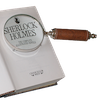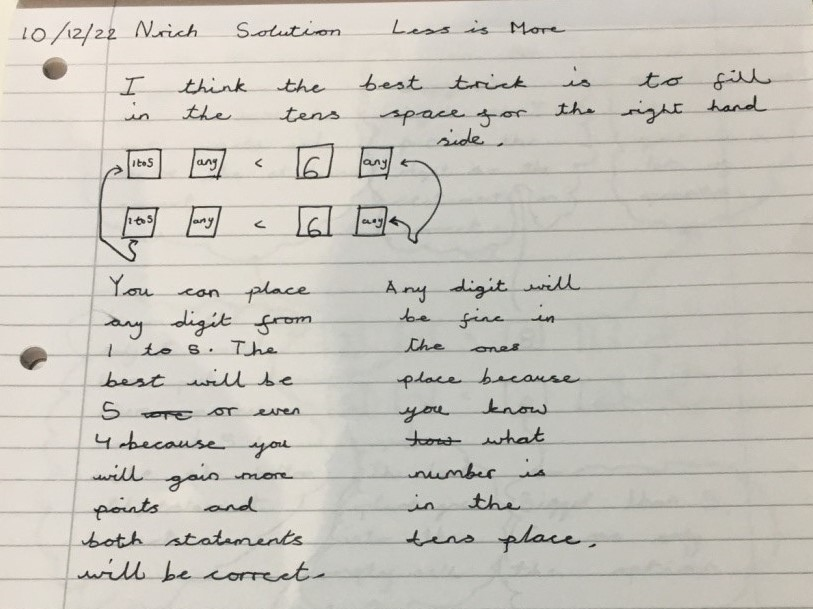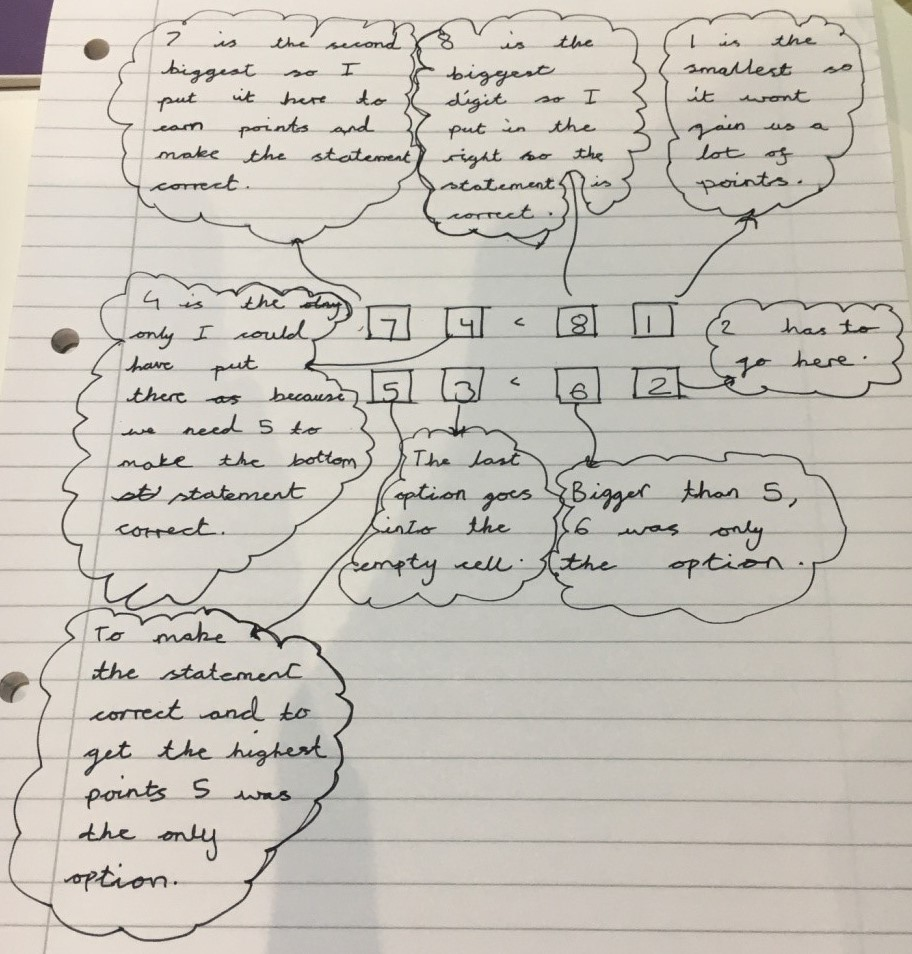#### You may also like### Number Detective

Follow the clues to find the mystery number.### Six Is the Sum

What do the digits in the number fifteen add up to? How many other numbers have digits with the same total but no zeros?### ABC

In the multiplication calculation, some of the digits have been replaced by letters and others by asterisks. Can you reconstruct the original multiplication?

# Less Is More

##### Age 7 to 11Challenge Level

Thank you to everybody who sent us their thoughts about this game. We received a couple of solutions for Version 1 and a lot of solutions for Version 2!

Version 1

Ilan from Twyford School in the UK considered which strategy worked best with a 1-6 dice:

The key to solving ‘less is more’ is to take a risk and put a large number on the smaller side. Here is an example of the perfect round to win:
65 < 66
65 < 66

This is because the left has to be smaller and it is quite unlikely to roll large numbers so this is quite a risky way of doing it.

This is a less risky round:
45 < 48
39 < 49

The risk to doing the first method is that if you have already put sixes in the first column there is a possibility that you will not get the number you want, in this case sixes and it will not work and you will be deducted a lot of points. In conclusion, I think that going in the middle (not too safe not too risky) so I think the best numbers to put on the right would be 51 or 56 or something along those lines.

Dhruv from St. Anne's RC Primary School in the UK also used a 1-6 dice, and they sent in a picture to explain their method. You can click on the picture to see the full-size solution:Good ideas, Ilan and Dhruv! It looks like you're both hoping to roll 6s or 5s. I wonder why Dhruv thinks it is best to fill in the tens spaces on the right-hand side first?

Version 2:

We received a lot of solutions from pupils at Halstead Prep School in England, explaining why the highest possible score is 127. Leticia sent in this explanation:

I wrote out out all the numbers from 1 to 8 and then picked 5, 6, 7 and 8. I then arranged them so that I had a 7 and a 5 on the left hand side. Then I put 8 and 6 on the right hand side. I then put the biggest two of the remaining numbers on the left hand side to get 74 and 53. Then I had 81 and 62 on the right hand side and that works. Then I added 74 and 53 and I got 127.

Christina agreed with Leticia's method of considering the largest digits first:

The highest score is 127. You should use 7 on the left and 8 on the right as it uses the less than sign. Then you fill in the units with the leftover lower digits. For the next calculation you would put 5 on the left and 6 on the right for the same reasons. You fill the units with the other numbers. This is how you get the highest score. For the units I put 3 and 4 on the left as they are the highest of the other numbers.

Amelie described a similar method:

The highest number possible is 127. The reason for this is:
You want the highest score on the left. So, use the largest numbers possible. 7 is less than 8 and 5 is less than 6. These are the largest tens digits possible. Next, we move on to the units digits. 1, 2, 3 and 4 remain. It doesn’t matter which numbers you use, as if the tens digit is larger, the number will be larger. To get the highest score possible, we will use 3 and 4. Our result is:
74 < 81
53 < 62
74 + 53 = 127

Well done to all of you for writing out your thinking so clearly. Thank you as well to Lila, Lottie, Ananya, Viva, Charlotte, Evie, Madeline and Emily who all sent in excellent justifications for why 127 is the maximum possible score.

We also received some similar solutions from children at Bishop's Castle Primary School in England. Lewis and Ryan sent in this explanation:

I put the highest number (8) in the top right tens column and the second highest number (7) in the opposite tens column because I knew I needed the largest total on the left hand side. The next highest number (6) I put in the tens column on the right hand side in the row below and the next highest number (5) in the tens on the left hand side. I took a similar approach with the position of the ones. This gives a top score of 127.

Lucy and Fynn had a similar strategy:

The 7 is smaller than 8 therefore you can use them on the same row in the tens. This strategy works for 6 and 5 as well. You can put 4 and 3 next to the 7 and 5 either way round in the units to make the largest possible correct numbers on the left hand side. The same strategy works for 1 and 2 on the right hand side. The highest score with digits 1-8 was 127.

Sid from Twyford School explained how they solved this problem by starting with the highest digits:

If you have the numbers 1,2,3,4,5,6,7 and 8, the way to get the best score would be looking at the highest numbers and going down to the lowest numbers. First you look at the eight, the eight cannot be in the first column in the left section as nothing else is bigger than eight. What you could then do is put 8 in the first column in the right section and seven in the first left section. That then means you now have the numbers 1,2,3,4,5 and 6. Like the 8 you can't put the 6 in the first left section so you would want to put it on the right first section under the 8, then you can have the number 5 in the left first one under the 7, you can then put 4 and 3 in the last places on the left and 2 and 1 on the right then your total would be 127.

Dhruv sent in this picture explaining their method:Thank you all for sending in these solutions!